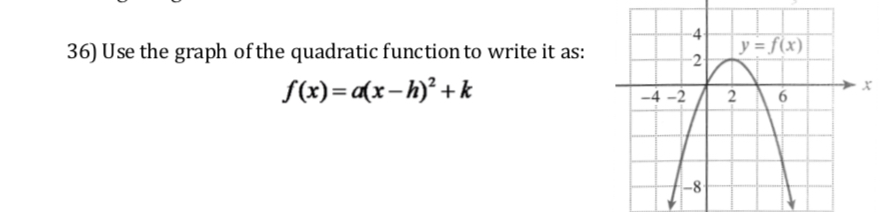# 4y-fx)36) Use the graph of the quadratic function to write it as:f(x)-a(x -h)'+k26-4-2

Question
9 viewshelp_outlineImage Transcriptionclose4 y-fx) 36) Use the graph of the quadratic function to write it as: f(x)-a(x -h)'+k 26 -4-2 fullscreen
check_circle

Step 1

The graph is downwards parabola whose equation is (y-k)=a(x-h)^2, where (h,k) is the vertex of parabola. Now, from graph , vertex is (2,2), that is h=2, k=2, so equation is shown on board.

Step 2

Also, it passes through (0,0). so it sa...

### Want to see the full answer?

See Solution

#### Want to see this answer and more?

Solutions are written by subject experts who are available 24/7. Questions are typically answered within 1 hour.*

See Solution
*Response times may vary by subject and question.
Tagged in

### Other